# Algebraic Diophantine equations

(diff) ← Older revision | Latest revision (diff) | Newer revision → (diff)

The work of Yu.V. Matiyasevich [a5], and J. Robinson [a8], (cf. Diophantine equations, solvability problem of) showed that in general there does not exist an algorithm by which it is possible to decide whether a given Diophantine equation over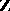has integral solutions. Neither does a general decision procedure exist for finding solutions of systems of such Diophantine equations in the ring of integers of any number field of finite degree over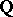.

Let(a1)

be a system of polynomial equations having integral coefficients. The ring of all algebraic integers,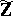, is defined to be the integral closure ofin an algebraic closure of(cf. also Algebraically closed field). In 1934, Th. Skolem suggested that the question: "Does there exist a decision procedure for finding solutions x1…xn of a system of polynomial equations in Z?" could be answered affirmatively.

More precisely, Skolem asked whether the elementary theory of the ring of all algebraic integersis decidable.

## Diophantine question.

In the early 1980s R. Rumely [a9] positively answered the Diophantine question, thereby showing that Hilbert's 10th problem (cf. Diophantine set) can be solved positively for. His proof built on work by D. Cantor and P. Roquette, [a1], who answered the corresponding question for systems of equations determining unirational varieties. The methods Rumely used for obtaining the general result are based on his capacity theory on algebraic curves over number fields.

The main point in the proof is the following Hasse local-global principle for Diophantine problems of the form (a1): Let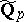be the algebraic closure of the-adic numbers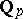and letbe the integral closure of the-adic integersin. The local-global principle for the ringasserts that if (a1) has solutions infor each, then there is a solution in.

## Decidability question.

By the decidability of the elementary theory of algebraically closed valued fields (cf. Model theory of valued fields), it follows that the elementary theory of, with valuation coming from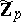, is decidable. For the given Diophantine problem (a1), it is only necessary to check whether there are solutions for finitely many critical primeswhich are effectively computable from the coefficients of (a1). Hence the solvability of arbitrary algebraic Diophantine equations inis decidable and Hilbert's 10th problem over the ringhas a positive answer.

The following steps go into the proof of the local-global principle: The result is first proved for curves, and then a Bertini-type induction argument is used to obtain the result for higher dimensions. For curves the proof is divided into two parts, a local/semi-local part and a local-to-global part. In each part a deep theorem is involved: For the local part it is the Rumely existence theorem for functions having prescribed poles and zeros lying in a given open subset of the set of all rational points of the curve over. The local-to-global part rests on Roquette's unit density approximation theorem. This theorem allows one to approximate arbitrarily closely at finitely many primes by an algebraic element which is a unit at all other primes in a suitable finite extension of.

The results above also hold for algebraic Diophantine equations defined over rings of integers of a global field, and, moreover, one is able to admit Archimedian primes and include rationality conditions at a finite set of primes. This general result is precisely stated below.

Letbe a global field and letbe an arbitrary prime of. An element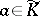, the algebraic closure, is called totally-adic overif for all embeddings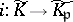, the imagelies in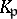, i.e. the primesplits completely in. If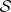is a finite set of primes of, then the set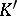of totally-adic elements overis the maximal extension in which all primes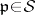split completely. It is a Galois extension of, and ifis empty it is taken to be the separable closure of. For any prime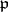one lets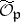be the integral closure of the completed unit ball in the algebraic closure of. Given a set of primesnot containing all primes of, one lets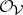be the set of all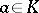that are contained infor all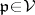. The integral closure inis denoted by.

## The local-global principle with rationality conditions.

Letbe a global field, equipped with a set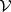of primes not containing all primes of. In addition, let a finite subsetbe given. Letbe a geometrically integral variety defined over, and let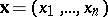be a finite family of rational functions ondefined over. Suppose that locally the set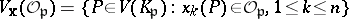contains a non-singular point for each, and that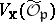is non-empty for. Then globally,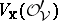is non-empty and it contains, moreover, non-singular points.

This theorem can be found in [a4], where the proof is given using methods from classical algebraic number theory and the theory of constant reductions. A proof using geometric methods can be found in [a6]. There, equivalent properties for separated schemes of finite type over appropriate Dedekind schemes are also given. Letting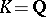and letting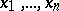be the coordinate functions for the variety determined by the system (a1) yields the local-global principle for algebraic Diophantine equations, whose variety is geometrically integral, with rationality conditions at a finite set of primes. The solvability of arbitrary systems of algebraic Diophantine equations whose variety is not geometrically integral can be effectively reduced to the geometrically integral case. An important question, which is still being investigated, concerns bounding the degree of integral solutions.

A direct geometric application of the local-global principle is to the existence of smooth curves of arbitrary genus over suitable number fields having good reduction everywhere. Another useful application is to the existence of finite morphisms to projective space. Letbe the spectrum of a Dedekind ring of integers of a global field and letbe a proper normal integral-scheme all fibres of which have dimension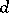. Then there exists a finite morphism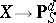. Another application is to the algebraic theory of.

## The algebraic theory of.

Using the local-global principle above, L. van den Dries [a2], and A. Prestel, J. Schmidt [a7], have independently shown that the elementary theory of the ringis decidable, answering the more general question posed by Skolem. In [a2], van den Dries extended the work of Rumely to an effective elimination of quantifiers for the elementary theory of, thus proving decidability for this theory. In [a7], this is done by studying the model theory of domains with radical extensions and proving model completeness of a certain class of such domains. Rumely's local-global principle is then used to show thatsatisfies the axioms of this class and so one obtains a complete effective axiomatization of the ring, which then also gives decidability.

How to Cite This Entry:
Algebraic Diophantine equations. Encyclopedia of Mathematics. URL: http://encyclopediaofmath.org/index.php?title=Algebraic_Diophantine_equations&oldid=14283
This article was adapted from an original article by B. Green (originator), which appeared in Encyclopedia of Mathematics - ISBN 1402006098. See original article